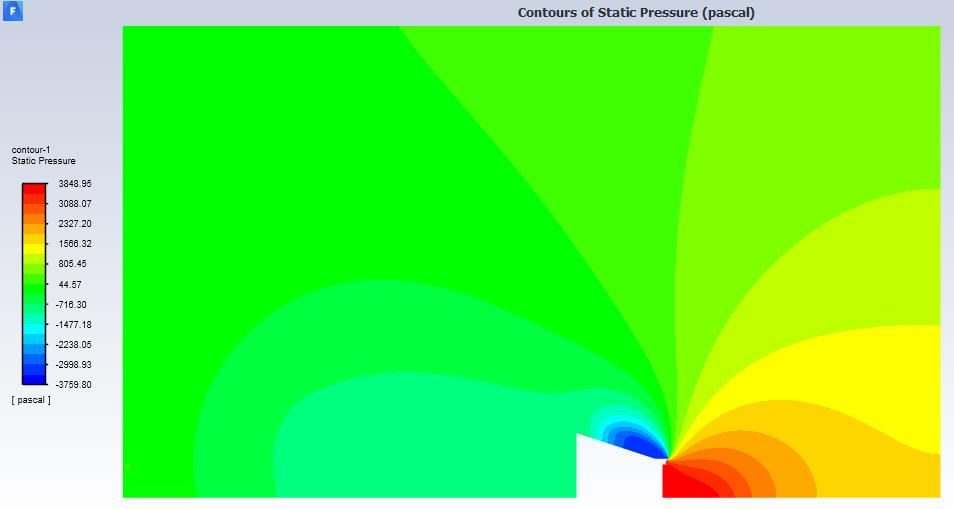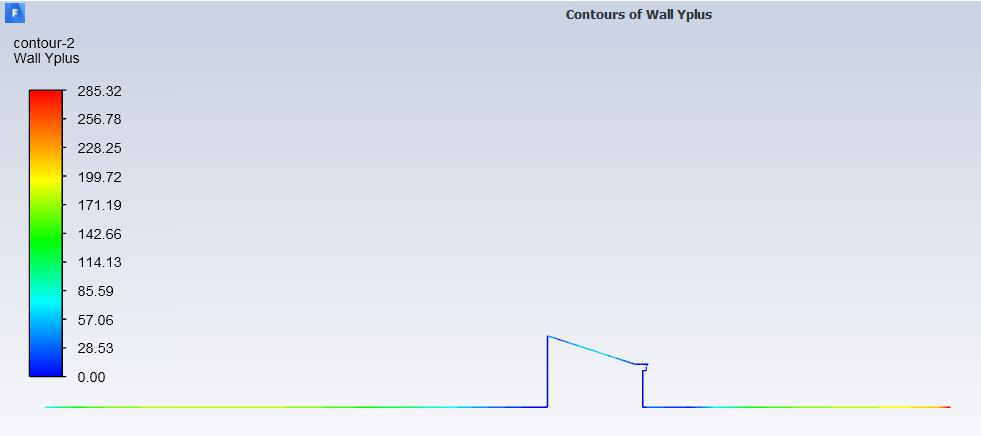## Fluids

Topics relate to Fluent, CFX, Turbogrid and more

•gabrielcamethru
Subscriber

I am trying to simulate category 5 hurricane winds on a roof using ANSYS 2019 R2 Fluent. My project is to design roof-edge devices that can reduce suction pressure on the roof.

I am concerned about the accuracy of the results I have been obtaining, particularly because I'm uncertain about the wall functions and the correctness of my inflation layers to represent the actual boundary layers.

As you can see in the pressure contour below, there is suction pressure in a flow separation region above the roof. Note that the right border of the air domain is the inlet, with air going left towards the house at 70 m/s.I have chosen to use non-equilibrium wall functions (NWF) for the near-wall treatment since I read that these wall functions consider flow separation. Use of NWF requires y+ values between 30 and 300 so that the centroids of the cells adjacent to walls may lie in the log layer of the boundary layer. I have assigned each edge a y+ value (ranging from 30 for Re = 500,000 and 300 for Re = 10^. When calculating the Re of each edge, I used corresponding edge length as the characteristic length.

To refine the mesh, I have been applying edge sizing and inflation layers on each edge other than the top border of the domain (the sky). I have been using equations for turbulent flow over a flat plate to calculate boundary layer thickness, δ, for each edge, as well as to calculate the wall shear stress needed to find y, the distance from the wall to the centroid of the cells in the adjacent layer. Obviously, these calculations can only give me very rough estimates for δ and y.

Then, I have used the first layer thickness inflation option and set the first layer height equal to 2y (so that the centroid of the cells in the first layer equals y), and each edge has 20 inflation layers whose combined thickness meet or exceed the calculated δ of that edge.

I've noticed that, except for the ground, my approach has not been accurate in keeping y+ values between 30 and 300.So, to sum up my questions:

1) Is it okay to be using non-equilibrium wall functions for this project? Or should another near-wall treatment be used?

2) How much should I concern myself with the y+ values? What tips would you recommend?

3) I was wondering how the reference values in setup change my results... Since my simulation is 2D, does changing Area or Depth do anything? Or do they only affect drag/lift coefficients (which I have no interest in) ?

Any other information or suggestions would also be greatly appreciated. Thank you beforehand

•Rob
Ansys Employee

Have a look at Enhanced Wall Treatment.

Also, think about the flow in a hurricane: is the velocity constant or does it fluctuate?